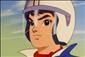# Arrays in Java: Determine Length of Arrays (Part-6)

When we declare an array in Java, it is treated as an object of an internal class that defines useful attributes and methods. Using these, we will be able to determine the length of the array and make a copy of its contents, in addition to other useful operations. We will now learn how to perform these operations.

The length field of the internal array class specifies the length of an array. The program implemented here illustrates how to use this field:

```public class MindStickArrayLengthTest{
public static void main(String[] args)              {                   final int SIZE = 5;                   int[ ] integerArray = new int[SIZE];                   float[ ] floatArray = { 5.0f, 3.0f, 2.0f, 1.5f };                   String[ ] weekDays = { 'Sunday', 'Monday', 'Tuesday', 'Wednesday',                                                    'Thursday', 'Friday', 'Saturday' };                   int[ ][ ] jaggedArray = { { 5, 4 }, { 10, 15, 12, 15, 18 }, { 6, 9, 10 },
{ 12, 5, 8, 11 } };
System.out.println('integerArray length: ' + integerArray.length);                   System.out.println('floatArray length: ' + floatArray.length);                   System.out.println('Number of days in a week: ' + weekDays.length);                   System.out.println('Length of jaggedArray: ' + jaggedArray.length);
int row = 0;                   for (int[ ] memberRow : jaggedArray) {                             System.out.println('\tArray length for row ' + ++row + ': '                                                + memberRow.length);
}
}

}```
##### In the main method, the program declares several arrays:

·   integerArray is a single-dimensional array consisting of five elements with default initial values.

·   floatArray is an array of floating-point numbers containing four initialized elements.

·  weekDays is an array of String objects initialized to the values of the days of the week.

·    jaggedArray is a nonrectangular initialized array.

To determine the length of each of these arrays, we use the syntax arrayName.length. The program output is shown here:

`integerArray length: 5floatArray length: 4Number of days in a week: 7Length of jaggedArray: 4Array length for row 1: 2Array length for row 2: 5Array length for row 3: 3Array length for row 4: 4`

·    The length of integerArray, where the elements are not explicitly initialized, is printed as 5.

·    the length of floatArray, where the elements are initialized using literals, is printed as 4.

·   and the length of array of Strings called weekDays, which is also initialized using literals, is printed as 7.

The interesting case here is determining the length of the jaggedArray. The expression jaggedArray.length returns the number of rows in this two-dimensional array. Each row is treated as an array; therefore, to determine the length of each row, we use the for-each loop discussed earlier to iterate through all the rows:

for (int[] memberRow : jaggedArray) {

Note how each element of the jaggedArray is considered an array of integers. The length of

each row is obtained using the expression memberRow.length.

Last updated:12/16/2017 4:42:57 AM#### David Miller